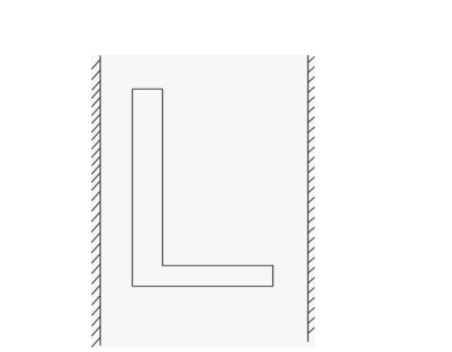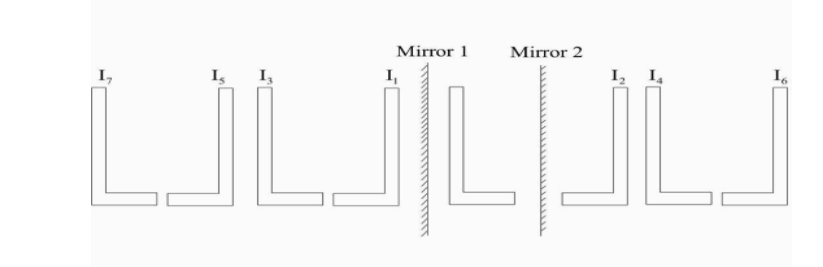Courses
Courses for Kids
Free study material
Free LIVE classes
More# An object shaped as L is placed between two parallel plane mirrors as shown. In the first seven closest images, how many laterally inverted images are formed?(A) 1 (B) 2 (C) 3 (D) 4Verified
289.2k+ views
Hint The phenomenon in plane mirrors in which our left is appeared as right and right is appeared as left is known as lateral inversion. The images formed by such inversion are known as laterally inverted images.

Step by step solution
Now first we will draw seven images of the object ( as per given in question)Due to the object L, Images $I_1$ and $I_2$ are formed.
Now image $\mathop I\nolimits_1$ acts as object 2 for mirror 2 and produces image $I_4$. Similarly, image $\mathop I\nolimits_2$acts as an object 1 for mirror 1 and produces image $I_3$. Now image $\mathop I\nolimits_3$ will act as an object for mirror 1 and produce images $\mathop I\nolimits_4$ and the process will continue till the formation of 7 images. When these images first act as an object they produce non – laterally inverted images.
Thus, by counting in figure, we conclude there are four laterally inverted images.
Now let us match the calculated and with the options given
1 :- This value does not match with the value that comes from calculation. Hence this option is not correct.
2 :- This value does not match with the value that comes from calculation. Hence this option is not correct.
3 :- this number matches with the value of non-lateral images produced and hence this option is not correct.
4 :- this value exactly matches with the number of laterally inverted images produced. Hence this option is correct.

Our required answer is d that is 4

Note
Plane mirror always produces virtual and erect images. The size of the image is always equal to that of the object. The image formed is as far behind the mirror as the object is in front of it. The images are laterally inverted.
Last updated date: 29th May 2023
Total views: 289.2k
Views today: 3.47k